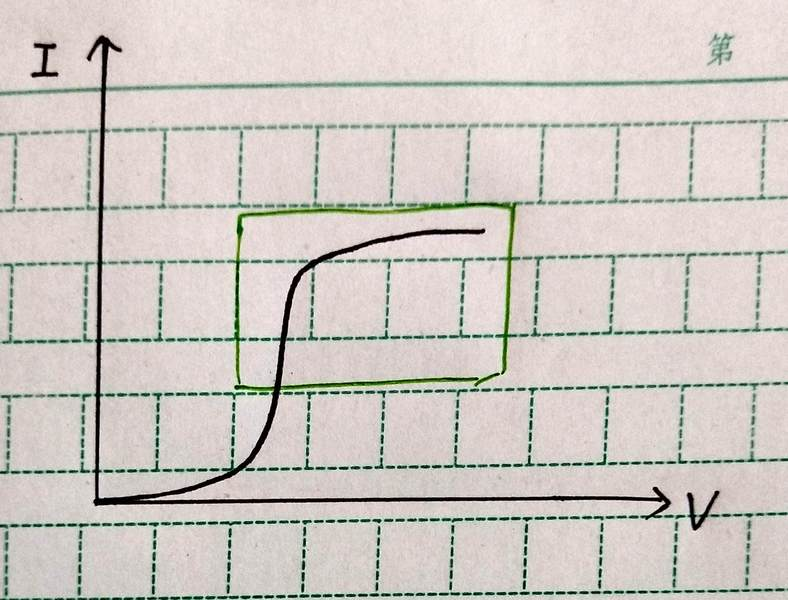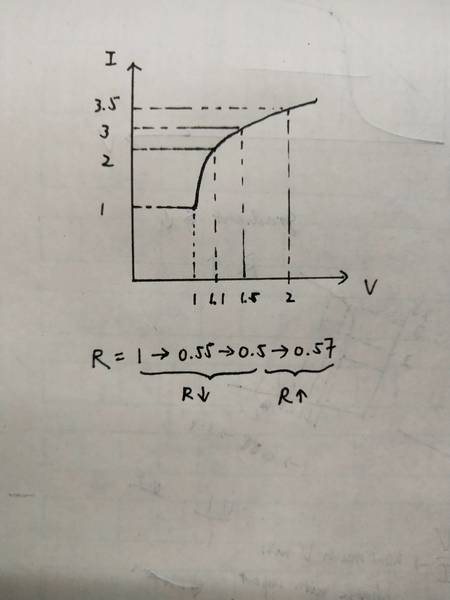# How to determine the change of resistance from I-V graph?1. Homework Statement

How to determine the change of R of a non-ohmic conductor from different parts of I-V graph?

R=V/I

## The Attempt at a Solution

For the part that passes through the origin, it is obvious that the reciprocal of the gradient, i.e. dV/dI, shows how R changes.
However, for the upper part(green part), since it does not passes though (0,0), the gradient only shows how I changes with V, but not how R changes with V.
I have tried to sub in numerical values as shown in the second picture, it seems that R varies in different ways even though I-V graph is concaving downwards.
Instead of sketching a separate R-V graph, are there any alternatives to tell the change in R directly from the graph?
Thanks a lot.

#### Attachments

SammyS
Staff Emeritus
Homework Helper
Gold Member
View attachment 231006
View attachment 231007
1. Homework Statement

How to determine the change of R of a non-ohmic conductor from different parts of I-V graph?

R=V/I

## The Attempt at a Solution

For the part that passes through the origin, it is obvious that the reciprocal of the gradient, i.e. dV/dI, shows how R changes.
However, for the upper part(green part), since it does not passes though (0,0), the gradient only shows how I changes with V, but not how R changes with V.
I have tried to sub in numerical values as shown in the second picture, it seems that R varies in different ways even though I-V graph is concaving downwards.
Instead of sketching a separate R-V graph, are there any alternatives to tell the change in R directly from the graph?
Thanks a lot.Hello @morrw1ndy . Welcome to PF !

Is this a homework problem or perhaps a part of some other school assignment?

Can you give some context here?Hello @morrw1ndy . Welcome to PF !

Is this a homework problem or perhaps a part of some other school assignment?

Can you give some context here?
Hi sir, this is a exam question which I may get the suggested answer 1 month later :)
It is a 2 mark explaination question, so I don't think detailed calculation is needed, and that's why I'm confused :)

kuruman
Homework Helper
Gold Member
2021 Award
Hi I have read through this thread... It seems that they did not arrive at a conclusionon?
Our syllabus makes it clear that R=V/I but not dV/dI.
So go by what your syllabus says and use R = V/I to find the resistance. Since you are asked to find the change in resistance, use ΔR = (V2/I2) - (V1/I1).

So go by what your syllabus says and use R = V/I to find the resistance. Since you are asked to find the change in resistance, use ΔR = (V2/I2) - (V1/I1).
Emm, but the question asks for a greneral trend, so I'm afraid that I won't be able to figure out all the delta V and delta I on the curve though

Merlin3189
Homework Helper
Gold Member
the question asks for a greneral trend, so I'm afraid that I won't be able to figure out all the delta V and delta I on the curve though
Surely you can see the trend by looking at the graph? That's why we draw graphs rather than using a table of data.
It's not clear where your graph came from. It could be drawn by hand from a table of data. It could have been drawn by someone trying to show you the general trend, based simply on their understanding of the physical situation. Maybe it was produced as the output from an electronic 'analyser' of some sort, but that would have been derived from data values from ADCs. In all cases the graph exists only because someone thought it would be easier to understand than the raw data.

So maybe you should not be trying to reconstruct the data from the graph - just look and see.
IMO all this argument about the definition of resistance is futile. You can find any number of examples where people use each of them. What is important here is to understand the physical behaviour of this (type of) element. And to realise that you have to be careful when people talk about resistance of non-linear elements.

kuruman
Homework Helper
Gold Member
2021 Award
Emm, but the question asks for a greneral trend, so I'm afraid that I won't be able to figure out all the delta V and delta I on the curve though
@Merlin3189 hit the nail on the head. If it's a general trend you want, then forget trying to get numbers from the graph. Just look at the sketch and provide a qualitative description of what it's saying to you.

I = V/R. This suggests that dI/dV = 1/R, and if R is constant, that is true. However, if R is not constant with respect to V, that clearly isn’t true. dI/dV is an interesting quantity, and it is called “differential resistance”, but don’t be fooled by the name. It is not the resistance. Looking at the slope of the graph is the wrong approach. You have a graph of I vs V. If you had a graph of V/I vs V, THEN you could look at the slope.

There are a few ways to approach this question. Graphically R at a point on the graph is the inverse of the slope of a line connecting that point to the origin, and you could reason about dR/dV that way. You could start with what kuruman said and calculate V/I at adjacent points. Or you could argue using calculus. Assume you know some functional form of I as a function of V: I(V). Start with the definition of R: R = V/I(V) and use the chain rule to express dR/dV